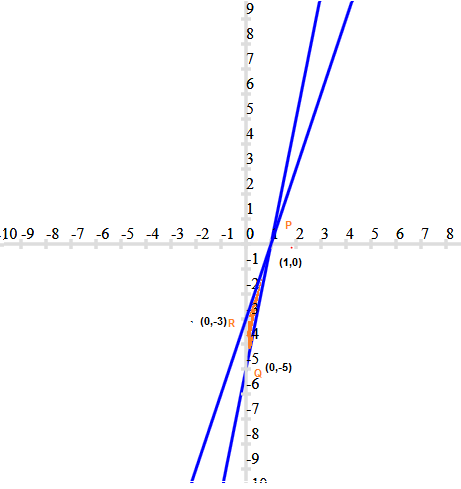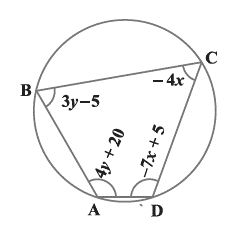# NCERT Solutions for Class 10th Maths:Linear Equations Exercise 3.7

In this page we have NCERT Solutions for Class 10th Maths:Linear Equations for Exercise 3.7 on page 68. Hope you like them and do not forget to like , social_share and comment at the end of the page.

## NCERT Solutions for Class 10 Maths Chapter 3 Exercise 3.7

Question 1
The ages of two friends Ani and Biju differ by 3 years. Ani’s father Dharam is twice as old as Ani and Biju is twice as old as his sister Cathy. The ages of Cathy and Dharam differ by 30 years. Find the ages of Ani and Biju.
Let x and y be the ages of Ani and Biju
x -y =3 (Ani is older than Biju)
Or y -x =3 (Biju is older than Ani)
Case 1 Ani is older than Biju
x -y =3   -(1)
Now Dharam age =2x
Cathy Age = y/2
Now
2x – y/2 =30
Or 4x -y=60 – (2)
Subtracting (1) from (2)
3x=57
x=19 year
So, y=16 year
Case 2 Biju is older than Ani

y -x =3 -(3)
Now Dharam age =2x
Cathy Age = y/2
Now
2x – y/2 =30
Or 4x -y=60 – (4)
3x=63
x=21 year
y=24 years

Question 2
One says, “Give me a hundred, friend! I shall then become twice as rich as you”. The other replies, “If you give me ten, I shall be six times as rich as you”. Tell me what is the amount of their (respective) capital? [From the Bijaganita of Bhaskara II]
[Hint: x + 100 = 2(y – 100), y + 10 = 6(x – 10)].
Let one have x Rs and other has y Rs, then
According to questions
x + 100 = 2(y – 100)
x-2y = -300 – (1)
y + 10 = 6(x – 10)
6x -y =70 -(2)
Multiplying (2) by 2 and subtracting from 1
11x =140 +300
x=40
Now substituting this in (1)
y=170

Question 3
A train covered a certain distance at a uniform speed. If the train would have been 10 km/h faster, it would have taken 2 hours less than the scheduled time. And, if the train were slower by 10 km/h; it would have taken 3 hours more than the scheduled time. Find the distance covered by the train.
Let x be the speed Km/h and y be the distance in Km and t (hour) be the time taken
Then
Speed = distance/time
x= y/t
or y=xt
Now Using the information that is given,
First Condition:
x+10= y/(t-2)
(x+10) (t-2) =d
xt -2x + 10 t-20 =d
Now d=xt, So
-2x+ 10 t=20 – (1)
Second condition:
x- 10= d/(t+3)
(x-10) (t+3) =d
xt-10t -30 +3x=d
3x-10t=30 --(2)
x= 50 km/hr.
Substituting this value in (1)
t=12 hour
Now d=xt = 600 km

Question 4
The students of a class are made to stand in rows. If 3 students are extra in a row, there would be 1 row less. If 3 students are less in a row, there would be 2 rows more. Find the number of students in the class.
Let the number of rows be x and the number of students in a row be x.
Total number of students:
= Number of rows x Number of students in a row
=xy
Using the information that is given,
First Condition:
Total number of students = (x– 1) (y + 3)
xy = ( x – 1 )(y + 3)
xy= xy – y + 3x – 3
3x – y = 3 – – – – – – – – – – – – – (1)
Second condition:
Total Number of students = (x+ 2 ) ( y – 3 )
Or xy = xy + 2y – 3x – 6
Or 3x – 2y = -6 – – – – – – – – – (2)
Subtracting equation (2) from (1)
y=9
By using the equation (1) we get,
3x – 9 =3
3x = 9+3 = 12
x = 4
Number of rows, x= 4
Number of students in a row, y = 9
Number of total students in a class =xy=> 4 x 9 = 36

Question 5
In a Δ ABC, ∠ C = 3 ∠ B = 2 (∠ A + ∠ B). Find the three angles.
Given,
∠C = 3 ∠B = 2(∠B + ∠A)
3∠B = 2 ∠A+2 ∠B
∠B=2 ∠A
2∠A – ∠B= 0 -- (i)
We know, the sum of all the interior angles of a triangle is 180°.
Thus, ∠ A +∠B+ ∠C = 180°
∠A + ∠B +3 ∠B = 180°
∠A + 4 ∠B = 180    ----(ii)
Multiplying equation (i) by 4 and adding (ii),we get
9 ∠A = 180°
∠A = 20°
Using this in equation (ii), we get
20O+ 4∠B = 180°
∠B = 40°
3∠B =∠C
∠C = 3 x 40 = 120°
Therefore, ∠A = 20°
∠B=40°
∠C = 120°

Question 6.
Draw the graphs of the equations 5x – y = 5 and 3x – y = 3. Determine the co-ordinates of the vertices of the triangle formed by these lines and the y axis.
For 5x -y =5
y=5x -5

 x 0 1 y -5 0
For 3x – y = 3
y= 3x-3

 x 0 1 y -3 0

Graphical representationFrom the above graph we can see that the triangle formed is $\Delta PQR$ (colored in red) by the lines and the y axis. Also, the coordinates of the vertices are P (1,0), Q (0, -5) and R (0, -3)
Question 7
Solve the following pair of linear equations:
(i) $px + qy = p -q$ ,$qx - py = p + q$
(ii) $ax + by = c$, $bx + ay = 1 + c$
(iii) $\frac {x}{a}- \frac {y}{b} =0$, $ax+ by= a^2 + b^2$
(iv) $(a - b)x + (a + b) y = a^2 - 2ab - b^2$
$(a + b)(x + y) = a^2 + b^2$
(v) $152x - 378y = - 74$
$-378x + 152y = - 604$
i) px + qy = p – q   -(1)
qx – py = p + q  -(2)
Multiplying p to equation (1)  and q to equation (2), Adding both , we get
p2   x + q2 x = p2 + q2
x=1
Substituting this value in equation (1)
y=-1

ii) ax + by = c   --(1)
bx + ay = 1 + c   ---(2)
Multiplying a to equation (i) and  b to equation (ii), and then subtracting (1) from (2) ,we get
a2 x - b2 x  =ac-b-bc
x = [c(a-b) -b]/(a2 -b2)
Putting this value in equation (1), we get
a[c(a-b) -b]/(a2 -b2)  +by =c
by = c - a[c(a-b) -b]/(a2 -b2
by = (ca2 -cb2 -ca2 +abc +ab)/ (a2 -b2)
x = [c(a-b) +a]/(a2 -b2)

iii)
(x/a) – (y/b) =0  => bx -ay=0  -(1)
ax+ by= a2 + b2      -(2)
Multiplying (1) by a and (2) by b and then subtracting (1) from (2)
a2 y   + b2 y =b( a2 + b2 )
y= b
Substituting this in (1)
x=a

iv)
(a + b) y + (a – b) x = a2− 2ab − b2 …………… (i)
(x + y)(a + b)  = a 2 + b2
(a + b) y + (a + b) x  = a2 + b2 ………………… (ii)
Subtracting equation (ii) from equation (i), we get
(a − b) x − (a + b) x = (a 2 − 2ab − b 2 ) − (a2 + b2 )
x(a − b − a − b) = − 2ab − 2b2
− 2bx = − 2b (b+a)
x = b + a
Substituting this value in equation (i), we get
(a + b)(a − b)  +y (a + b)  = a2− 2ab – b2
a2 − b2 + y(a + b)  = a2− 2ab – b2
(a + b) y = − 2ab
y=-2ab/(a+b)

v)
152x − 378y = − 74
76x − 189y = − 37
x=(189y-37)/76………(i)
− 378x + 152y = − 604
− 189x + 76y = − 302 ………….. (ii)
Using the value of x in equation (ii), we get
−189[(189y−37)/76]+76y=−302
− (189) 2 y + 189 × 37 + (76) 2 y = − 302 × 76
189 × 37 + 302 × 76 = (189) 2 y − (76) 2y
6993 + 22952 = (189 − 76) (189 + 76) y
29945 = (113) (265) y
y = 1
Using equation (i), we get
x =(189-37)/76
x=152/76 =2

Question 8.Find the angles of the cyclic quadrilateral.
It is know that the sum of the opposite angles of a cyclic quadrilateral is 180o
Thus, we have
∠C +∠A = 180
4y + 20− 4x = 180
− 4x + 4y = 160
x − y = − 40 ……………(1)
And, ∠B + ∠D = 180
3y − 5 − 7x + 5 = 180
− 7x + 3y = 180 ………..(2)
Multiplying 3 to equation (1), we get
3x − 3y = − 120 ………(3)
Adding equation (2) to equation (3), we get
− 7x + 3x = 180 – 120
− 4x = 60
x = −15
Substituting this value in equation (i), we get
x − y = − 40
-y−15 = − 40
y = 40-15
= 25
∠A = 4y + 20 = 20+4(25)  = 120°
∠B = 3y − 5 = − 5+3(25)  = 70°
∠C = − 4x = − 4(− 15) = 60°
∠D =  5-7x
∠D=  5− 7(−15) = 110°

## Summary

1. NCERT Solutions for Class 10 Maths Chapter 3 Linear Equations Exercise 3.7 has been prepared by Expert with utmost care. If you find any mistake.Please do provide feedback on mail.
2. This chapter 3 has total 7 Exercise 3.1 ,3.2,3.3,3.4,3.5,3.6 and 3.7. This is the Seventh exercise in the chapter.You can explore previous exercise of this chapter by clicking the link belowGo back to Class 10 Main Page using below links

### Practice Question

Question 1 What is $1 - \sqrt {3}$ ?
A) Non terminating repeating
B) Non terminating non repeating
C) Terminating
D) None of the above
Question 2 The volume of the largest right circular cone that can be cut out from a cube of edge 4.2 cm is?
A) 19.4 cm3
B) 12 cm3
C) 78.6 cm3
D) 58.2 cm3
Question 3 The sum of the first three terms of an AP is 33. If the product of the first and the third term exceeds the second term by 29, the AP is ?
A) 2 ,21,11
B) 1,10,19
C) -1 ,8,17
D) 2 ,11,20